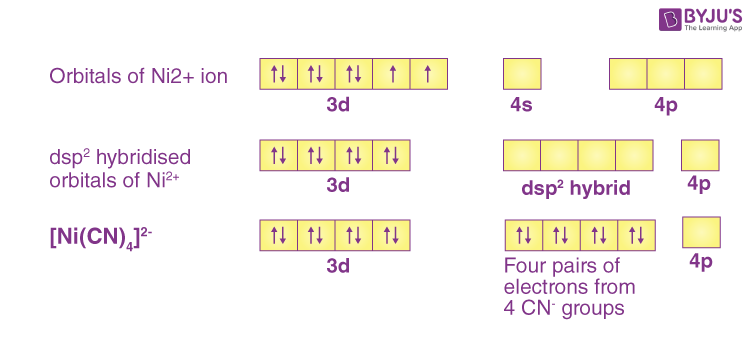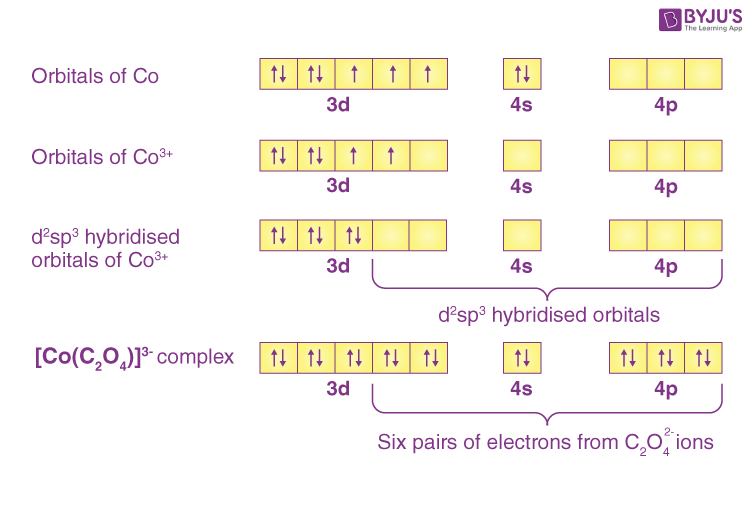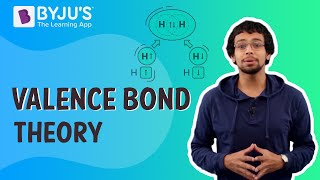Jet Set Go! All about Aeroplanes Jet Set Go! All about Aeroplanes

# Valence Bond Theory Questions

Many theories have been proposed to explain the nature of bonding in coordination compounds, including the Valence Bond Theory (VBT).The Valence Bond Theory was developed to explain chemical bonding using the quantum mechanics method.

 Definition: According to these theory under the influence of ligands, a metal atom or ion can use its (n-1)d, ns, np or ns, np, nd orbitals for hybridisation, yielding a set of equivalent orbitals of definite geometry such as octahedral, tetrahedral, square planar, and so on. These hybridised orbitals are permitted to overlap with ligand orbitals capable of donating electron pairs for bonding.

## Valence Bond Theory Chemistry Questions with Solutions

Q-1: Explain the nature of bonding in [Ni(CN)4]2- on the basis of valence bond theory.

Answer: In the complex [Ni(CN)4]2-, nickel is in +2 oxidation state and has the electronic configuration 3d 8. A cyanide ion delivers a pair of electrons to each of the hybridised orbitals. The ligand cyanide (CN) is a strong field ligand. Therefore, it will cause pairing of electrons. The hybridisation scheme is as shown in below diagram:The absence of unpaired electrons indicates that the compound is diamagnetic.

Q-2: Which of the following is correct for s-orbital?

1. Directional
2. Non directional
3. Has zero angular momentum
4. Electron density is unequal

Explanation: The probability of finding an electron in all directions at a given distance from the nucleus is the same which makes s-orbital non-directional and spherically symmetrical.

The angular momentum for any orbital can be calculated using the below formula:

$$\begin{array}{l}L=\sqrt{l(l+1)} \frac{h}{2\pi}\end{array}$$

For s-orbital, l=0

This makes angular momentum L=0.

Q-3: Which of the following is correct for [Co(C2O4)3]3- ?

1. dsp2, square planar
2. dsp2, tetrahedral
3. sp3d2, octahedral
4. d2sp3, octahedral

Explanation: In [Co(C2O4)3]3-, Co is in +3 oxidation state and has electronic configuration 3d6. The ligand oxalate ion is a strong field ligand which causes pairing of electrons. The hybridization scheme is shown below:Because the hybridisation is d2sp3,the complex is octahedral.

Q-4: Calculate the spin only magnetic moment value for [Mn(Br)4]2-. Also predict the geometry of the complex ion.

In the complex [Mn(Br)4]2-, Mn has an electronic configuration of 3d5 and is in the +2 oxidation state. According to the spectrochemical series, bromide ion (ligand) is a weak field ligand. As a result, all five d-orbital electrons will remain unpaired, giving n = 5.

The spin only magnetic moment of a complex can be calculated using the formula:

$$\begin{array}{l}\mu = \sqrt{n(n+2}\end{array}$$

Where, n= number of unpaired electrons.

$$\begin{array}{l}\mu = \sqrt{n(n+2}= \sqrt{5(5+2}=5.9 BM\end{array}$$

Since the coordination number of Mn2+ ion in the complex ion is 4, it will be either tetrahedral (sp3 hybridisation) or square planar (dsp2 hybridisation). However, because the complex ion has a magnetic moment of 5.9 BM, it should be tetrahedral in shape rather than square planar due to the presence of five unpaired electrons in the d orbitals.

Q-5: The orbitals involved in hybridisation in PtCl42- is

1. 6s, 6px,6py,6pz
2. 5s, 5px, 5py,5pz
3. 6s, 6px, 6py, 5dx2-y2
4. 5s, 5px, 5py, 4dx2-y2

Answer: c) 6s, 6px, 6py, 5dx2-y2

Explanation: The coordination number of PtCl42- is 4. Coordination number 4 allows for either square planar or tetrahedral shapes. However, when metals from the 4d and 5d series are present, all ligands act as strong field ligands. Strong field strength usually results in a square planar geometry. Because Pt is in the 5d series, PtCl42- is square planar.

In square planar geometry, the orbitals involved are 6s, 6px, 6py, 5dx2-y2

Q-6: Which of the following is true for (X) [Ru(ox)3]3- and (Y) [Fe(ox)3]3-

1. X has low spin and Y has high spin configuration
2. X has high spin and Y has low spin configuration
3. Both X and Y are high spin
4. Both X and Y are low spin

Answer: a) X has low spin and Y has high spin configuration

Explanation: Ru is in the oxidation state +3 and has the d-electronic configuration 4d5 in X) [Ru(ox)3]3-.When Ru is in the +3 oxidation state, oxalate acts as a strong field ligand. A low spin complex is produced by a strong field strength.

[Fe(ox)3]3-, on the other hand, has Fe in the oxidation state of +3 and a d-electronic configuration of 4d5. When iron is in the +3 oxidation state, oxalate acts as a weak field ligand. A weak field strength produces a high spin complex.

Note: When the metal is in the +3 oxidation state and belongs to the 4d/5d series, oxalate acts as a strong field ligand. Except for cobalt, it acts as a weak field in the 3d series for all metals in the +3 oxidation state.

Q-7: The magnetic moment of [Ru(H2O)6]2+ corresponds to the presence of

1. Four unpaired electrons
2. Zero unpaired electrons
3. Two unpaired electrons
4. Three unpaired electrons

Explanation: Ru is in the +2 oxidation state and has an electronic configuration of 4d6 in the given complex. When a metal belongs to the 4d/5d series, all ligands, regardless of oxidation state, act as strong field ligands. As a result of water acting as a strong field ligand, all electrons in 4d6 will pair, leaving zero unpaired unpaired electrons. As a result, there is no magnetic moment.

Q-8: In the complex [Cr(NH3)6]Br3,chromium has the correct distribution of 3d electrons as

1. 3d1xy, 3d1yz, 3d1x2
2. 3d1xy, 3d1yz, 3d1xz
3. 3d1xz, 3d1x2-y2 , 3d1yz
4. $$\begin{array}{l}3d^{1}_{x^{2}-y^{2}}, 3d_{x^{2}}^{1}, 3d^{1}_{xz}\end{array}$$

Explanation: In the complex [Cr(NH3)6]Br3, chromium has electronic configuration of 3d3 with + 3 oxidation state. The distribution of electrons in the d-orbital is shown below:

Q-9: For [Ni(CO)4] and K2[Ni(CN)4], which property is the same for the given pairs.

1. Oxidation state of central atom
2. Magnetic moment
3. Electronic configuration
4. Shape

Explanation: Ni has zero and +2 oxidation states in the given complexes, with 3d10 and 3d8 electron configurations, respectively.

The magnetic moment is known to be proportional to the number of unpaired electrons. CO and cyanide ligands are both strong field ligands that cause electrons to pair up in the 3d orbital. As a result, both complexes have zero unpaired electrons and thus have the same magnetic moment value.

Q-10: Consider the following reaction

$$\begin{array}{l}K_{2}[Ni(CN)_{4}] \overset{Na/liqNH_{3}}{\rightarrow} K_{4}[Ni(CN)_{4}]\end{array}$$

Will there be any change in magnetic moment?

Explanation: In both the complexes Ni has the same number of unpaired electrons equal to zero. Therefore no change in magnetic moment.

Note: Cyanide is a strong field ligand which causes pairing.

Q-11: State the limitations of Valence Bond theory.

Answer: While the VB theory explains the formation, structures, and magnetic behaviour of coordination compounds to a large extent, it has the following shortcomings:

(i) It is predicated on a number of assumptions.

(ii) No quantitative interpretation of magnetic data is provided.

(iii) It does not account for the colour of coordination compounds.

(iv) It provides no quantitative interpretation of thermodynamics or coordination compound kinetic stabilities.

(v) It cannot tell the difference between weak and strong ligands.

Q-12 Among the following aqua complex, a low spin complex is

1. [Ti(H2O)6]3+
2. [Co(H2O)6]3+
3. [Co(H2O)6]2+
4. None of the above

Explanation: Co is in the +3 oxidation state with an electronic configuration of 3d6 in [Co(H2O)6]3+. When cobalt is in a +3 OS state, o-donors (such as H2O) act as a strong field ligand. As a result, the complex has a low spin value.

When cobalt is in a +2 OS state, o-donors (such as H2O) act as a weak field ligand.In complex [Co(H2O)6]2+, water acts as a weak field ligand with Co in +2 oxidation state resulting in high spin complex.

In the complex [Ti(H2O)6]3+, Ti is in +1 oxidation state with 3d1 as electronic configuration. There is no need to check field strength between 3d1-3d3. Every complex has a high spin.

Note: [Co(H2O)6]3+ is the only aqua complex which is low spin.

Q-13: For the complex A: [Ag(CN)4]-1 and B: [Au(CN)4]2-, which statement is true?

1. Both are paramagnetic
2. Bothe are diamagnetic
3. A is paramagnetic and B is diamagnetic
4. A is diamagnetic and B is paramagnetic

Answer: d) A is diamagnetic and B is paramagnetic

Explanation: A diamagnetic complex has zero unpaired electrons and a paramagnetic has unpaired electrons.

[Ag(CN)4]-1 has silver in +3 oxidation state with 4d8 as electronic configuration. All electrons get paired up due to the strong field strength of cyanide ion.Hence, a diamagnetic complex.

[Au(CN)4]2- has gold in an oxidation state of +2 and an electronic configuration of 5d9. Despite the fact that cyanide is a strong field ligand, an electron is left unpaired even after pairing. As a result, making it a paramagnetic complex.

Q-14: The structure of the complexes [Cu(NH3)4](ClO4)2 and [Cu(NH3)4](ClO4) in the solution respectively are:

1. Square planar and tetrahedral
2. Octahedral and square pyramidal
3. Octahedral and trigonal pyramidal
4. Tetrahedral and square planar

Answer: a) Square planar and tetrahedral

Explanation: In the complex [Cu(NH3)4](ClO4)2, copper is in +2 oxidation state with electronic configuration of 3d9. Upon filing electrons, one electron will remain unpaired and it gets transferred from 3d to 4p. One 3d, one 4s and two 4p orbital gets hybridised to give dsp2 hybridisation.(square planar)

In the complex [Cu(NH3)4](ClO4), copper is in +1 oxidation state with electronic configuration of 3d10.One 4s and three 4p orbital gets hybridised to give sp3 hybridisation.(tetrahedral)

Q-15: Why are inner orbital complexes preferred over outer orbital complexes?

Answer: Inner orbital complexes are made up of metal atoms that use inner shell d orbitals((n-1)d) to hybridise. Outer orbital complexes are made up of metal atoms that use the outermost shell d orbitals(nd) for hybridization.

The energy gap between the ns and (n-1)d is less as compared to ns and nd orbitals of a metal atom.Therefore, inner orbital complexes are preferred over outer orbital complexes.

## Practise Questions on Valence Bond Theory

Q-1: The minimum number of monodentate ligand required for the formation of square planar and tetrahedral complex is

1. 2
2. 3
3. 4
4. 6

Q-2: Cuprammonium ion has ___________ shape.

1. Octahedral
2. Tetrahedral
3. Trigonal
4. Square planar

Q-3: The square planar geometry is based on

1. Z-exclusion principle
2. Electroneutrality principle
3. None of the above
4. Pauli exclusion principle

Q-4: The zero magnetic moment of octahedral K2NiF6 is due to

1. Low spin d6Ni(IV) complex
2. Low spin d8Ni(IV) complex
3. High spin d8Ni(IV) complex
4. High spin d6Ni(IV) complex

Q-5: For the central metal atom in a complex, coordination number is

1. The number of ligands bonded by pi-bonds that surround a metal ion.
2. The number of ligands bonded by sigma-bonds that surround a metal ion.
3. The number of ligands bonded by pi-bonds and sigma bonds that surround a metal ion.
4. The number of only neutral ligands bonded to the metal ion

Click the PDF to check the answers for Practice Questions.

## Recommended Videos

#### Valence Bond Theory#### Valence Bond Theory (VBT), Orbital Overlap: Chemical Bonding